# Electronics and Communication Engineering - Exam Questions Papers

31.

The probability density function (PDF) of a random variable X is as shown below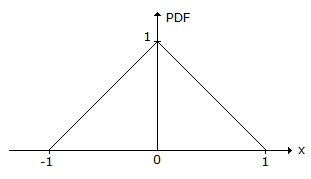The corresponding cumulative distribution function (CDF) has the form

 A.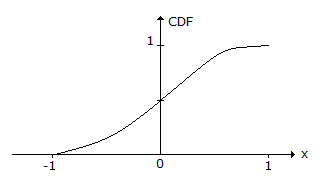B.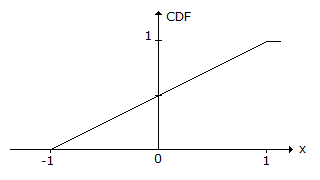C.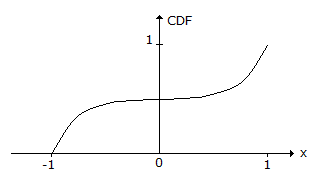D.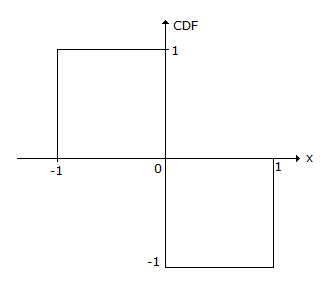Answer: Option A

Explanation:

Cumulative distribution function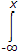(Pdf) dx

Then when we integrate the line from (-1, 0) to (0, 1) we get a parabolic curve.

The maximum value of Pdf can be 1.

32.

The system with given pole-zero diagram is __________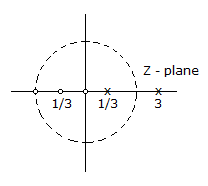A. Causal B. Anti-causal C. Both sided D. Cannot be determined

Answer: Option D

Explanation:

Z transform for the pole zero plot will be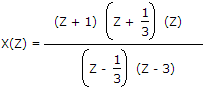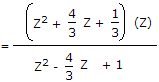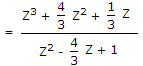Degree of numerator > degree of denominator. Also ROC is not defined.

Hence response of the system cannot be determined.

33.

For the waveform shown below, the slew rate and full power bandwidth with respect to op-amp will be respectively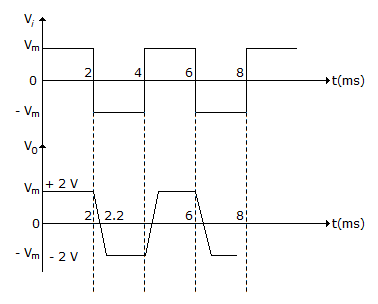A. 0.02 V/μsec, 1.59 KHz B. 0.04 V/μsec, 1.59 KHz C. 0.02 V/μsec, 3.58 KHz D. 0.02 V/μsec, 1.59 KHz

Answer: Option A

Explanation: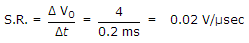Full power bandwidth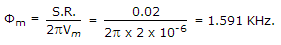34.

In the derivation of expression for peak percent overshoot,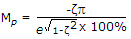, Which one the following conditions is NOT required?

 A. System is linear and time invariant B. The system transfer function has a pair of complex conjugate poles and no zeros. C. There is no transportation delay in the system. D. The system has zero initial conditions.

Answer: Option C

Explanation: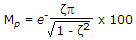.

35.

The impulse response h(t) of a linear invariant continuous time system is given by h(t) = exp (- 2t) u(t), where u(t) denotes the unit step function. The output of this system to the sinusoidal input x(t) = 2cos(2t) for all time t, is

 A. 0 B. 2-0.25 cos (2t - 0.125p) C. 2-0.5 cos (2t - 0.125p) D. 2-0.5 cos (2t - 0.25p)

Answer: Option D

Explanation:

When input is sinusoidal then output is also sinusoidal with same frequency but amplitude and phase changes. Thus amplitude is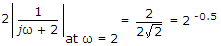Phase is tan-1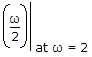= 0.25 p

Thus y(t) = 2-0.5 cos(2t - 0.25p).

#### Current Affairs 2021

Interview Questions and Answers